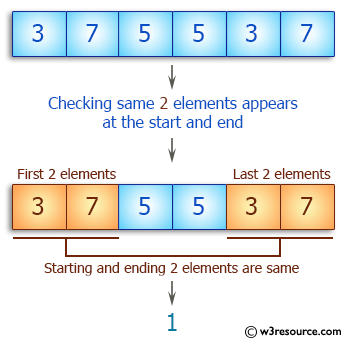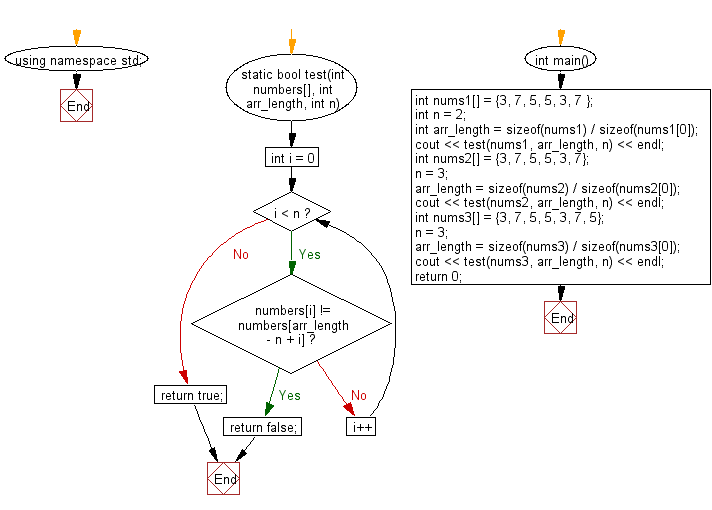﻿ C++ : Check an array starts and ends with the same elements# C++ Exercises: Check a given array of integers and return true if the specified number of same elements appears at the start and end of the given array

## C++ Basic Algorithm: Exercise-114 with Solution

Write a C++ program to check a given array of integers and return true if the specified number of same elements appears at the start and end of the given array.

Sample Solution:

C++ Code :

``````#include <iostream>

using namespace std;

static bool test(int numbers[], int arr_length, int n)
{

for (int i = 0; i < n; i++)
{
if (numbers[i] != numbers[arr_length - n + i])
{
return false;
}
}

return true;
}

int main()
{
int nums1[] = {3, 7, 5, 5, 3, 7 };
int n = 2;
int arr_length = sizeof(nums1) / sizeof(nums1);
cout << test(nums1, arr_length, n) << endl;
int nums2[] = {3, 7, 5, 5, 3, 7};
n = 3;
arr_length = sizeof(nums2) / sizeof(nums2);
cout << test(nums2, arr_length, n) << endl;
int nums3[] = {3, 7, 5, 5, 3, 7, 5};
n = 3;
arr_length = sizeof(nums3) / sizeof(nums3);
cout << test(nums3, arr_length, n) << endl;
return 0;
}
``````

Sample Output:

```1
0
1
```

Pictorial Presentation:Flowchart:C++ Code Editor: